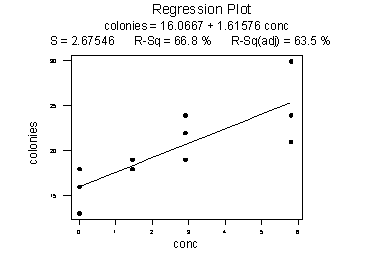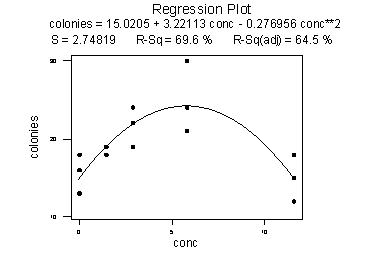# 10.10 - Other Regression Pitfalls

Overfitting

When building a regression model, we don't want to include unimportant or irrelevant predictors whose presence can overcomplicate the model and increase our uncertainty about the magnitudes of the effects for the important predictors (particularly if some of those predictors are highly collinear). Such "overfitting" can occur the more complicated a model becomes and the more predictor variables, transformations, and interactions are added to a model. It is always prudent to apply a sanity check to any model being used to make decisions. Models should always make sense, preferably grounded in some kind of background theory or sensible expectation about the types of associations allowed between variables. Predictions from the model should also be reaosnable (over-complicated models can give quirky results that may not reflect reality).

Excluding Important Predictor Variables

However, there is potentially greater risk from excluding important predictors than from including unimportant ones. The linear association between two variables ignoring other relevant variables can differ both in magnitude and direction from the association that controls for other relevant variables. Whereas the potential cost of including unimportant predictors might be increased difficulty with interpretation and reduced prediction accuracy, the potential cost of excluding important predictors can be a completely meaningless model containing misleading associations. Results can vary considerably depending on whether such predictors are (inappropriately) excluded or (appropriately) included. These predictors are sometimes called confounding or lurking variables, and their absence from a model can lead to incorrect decisions and poor decision-making.

Extrapolation

"Extrapolation" beyond the "scope of the model" occurs when one uses an estimated regression equation to estimate a mean µY or to predict a new response ynew for x values not in the range of the sample data used to determine the estimated regression equation. In general, it is dangerous to extrapolate beyond the scope of model. The following example illustrates why this is not a good thing to do.

Researchers measured the number of colonies of grown bacteria for various concentrations of urine (ml/plate). The scope of the model — that is, the range of the x values — was 0 to 5.80 ml/plate. The researchers obtained the following estimated regression equation:Using the estimated regression equation, the researchers predicted the number of colonies at 11.60 ml/plate to be 16.0667 + 1.61576(11.60) or 34.8 colonies. But when the researchers conducted the experiment at 11.60 ml/plate, they observed that the number of colonies decreased dramatically to about 15.1 ml/plate:The moral of the story is that the trend in the data as summarized by the estimated regression equation does not necessarily hold outside the scope of the model.

Missing Data

Real-world datasets frequently contain missing values, so that we do not know the values of particular variables for some of the sample observations. For example, such values may be missing because they were impossible to obtain during data collection. Dealing with missing data is a challenging task. Missing data has the potential to adversely affect a regression analysis by reducing the total usable sample size. The best solution to this problem is to try extremely hard to avoid having missing data in the first place. When there are missing values that are impossible or too costly to avoid, one approach is to replace the missing values with plausible estimates, known as imputation. Another (easier) approach is to consider only models that contain predictors with no (or few) missing values. This may be unsatisfactory, however, because even a predictor variable with a large number of missing values can contain useful information.

Power and Sample Size

In small datasets, a lack of observations can lead to poorly estimated models with large standard errors. Such models are said to lack statistical power because there is insufficient data to be able to detect significant associations between the response and predictors. So, how much data do we need to conduct a successful regression analysis? A common rule of thumb is that 10 data observations per predictor variable is a pragmatic lower bound for sample size. However, it is not so much the number of data observations that determines whether a regression model is going to be useful, but rather whether the resulting model satisfies the LINE conditions. In some circumstances, a model applied to fewer than 10 data observations per predictor variable might be perfectly fine (if, say, the model fits the data really well and the LINE conditions seem fine), while in other circumstances a model applied to a few hundred data points per predictor variable might be pretty poor (if, say, the model fits the data badly and one or more conditions are seriously violated). For another example, in general we’d need more data to model interaction compared to a similar model without the interaction. However, it is difficult to say exactly how much data would be needed. It is possible that we could adequately model interaction with a relatively small number of observations if the interaction effect was pronounced and there was little statistical error. Conversely, in datasets with only weak interaction effects and relatively large statistical error, it might take a much larger number of observations to have a satisfactory model. In practice, we have methods for assessing the LINE conditions, so it is possible to consider whether an interaction model approximately satisfies the assumptions on a case-by-case basis. In conclusion, there is not really a good standard for determining sample size given the number of predictors, since the only truthful answer is, “It depends.” In many cases, it soon becomes pretty clear when working on a particular dataset if we are trying to fit a model with too many predictor terms for the number of sample observations (results can start to get a little odd and standard errors greatly increase). From a different perspective, if we are designing a study and need to know how much data to collect, then we need to get into sample size and power calculations, which rapidly become quite complex. Some statistical software packages will do sample size and power calculations, and there is even some software specifically designed to do just that. When designing a large, expensive study, it is recommended that such software be used or to get advice from a statistician with sample size expertise.